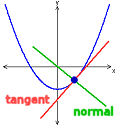Go to contentTo find the gradient of a tangent to a curve y=f(x) at a given point x=a:
firstly differentiate the equation of the curve in order to find \displaystyle\frac{dy}{dx} ,
secondly substitute into this expression the value x=a.

## Summary/Background

If y = ax^n then \displaystyle\frac{dy}{dx} = anx^{n-1} and if y = a then \displaystyle \frac{dy}{dx} = 0, where a is a constant.
These work for all values of n, positive or negative, decimal or fraction.You can get a better display of the maths by downloading special TeX fonts from jsMath. In the meantime, we will do the best we can with the fonts you have, but it may not be pretty and some equations may not be rendered correctly.

## Glossary

### constant

a value in a formula or equation that cannot change.

### differentiate

to find the derivative of a function

### equation

A statement that two mathematical expressions are equal.

### fraction

A ratio of two numbers or polynomials.

The slope of a line; the angle of its inclination to the horizontal.

### tangent

1. The trigonometrical function defined as opposite/adjacent in a right-angled triangle.
2. A straight line that touches a curve at one point.

### union

The union of two sets A and B is the set containing all the elements of A and B.

### work

Equal to F x s, where F is the force in Newtons and s is the distance travelled and is measured in Joules.

Full Glossary List

## This question appears in the following syllabi:

SyllabusModuleSectionTopicExam Year
AP Calculus AB (USA)3DifferentiationCurves-
AP Calculus BC (USA)3DifferentiationCurves-
AQA A-Level (UK - Pre-2017)C1DifferentiationCurves-
AQA AS Maths 2017Pure MathsDifferentiationTangents and Normals-
AQA AS/A2 Maths 2017Pure MathsDifferentiationTangents and Normals-
CBSE XII (India)CalculusApplications of DerivativesTangents and normals-
CCEA A-Level (NI)C1DifferentiationCurves-
CIE A-Level (UK)P1DifferentiationCurves-
Edexcel A-Level (UK - Pre-2017)C1DifferentiationCurves-
Edexcel AS Maths 2017Pure MathsDifferentiationTangents and Normals-
Edexcel AS/A2 Maths 2017Pure MathsDifferentiationTangents and Normals-
I.B. Higher Level6DifferentiationCurves-
I.B. Standard Level6DifferentiationCurves-
Methods (UK)M8DifferentiationCurves-
I.B. (MSSL)7DifferentiationCurves-
OCR A-Level (UK - Pre-2017)C1DifferentiationCurves-
OCR AS Maths 2017Pure MathsApplying DifferentiationTangents and Normals-
OCR MEI AS Maths 2017Pure MathsApplying DifferentiationTangents and Normals-
OCR-MEI A-Level (UK - Pre-2017)C2DifferentiationCurves-
Pre-U A-Level (UK)4DifferentiationCurves-